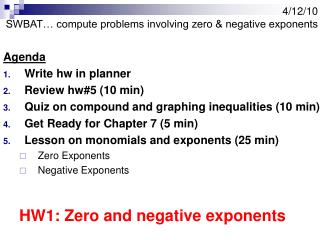DownloadDownload Presentation4/12/10 SWBAT… compute problems involving zero & negative exponents

# 4/12/10 SWBAT… compute problems involving zero & negative exponents

Download Presentation## 4/12/10 SWBAT… compute problems involving zero & negative exponents

- - - - - - - - - - - - - - - - - - - - - - - - - - - E N D - - - - - - - - - - - - - - - - - - - - - - - - - - -
##### Presentation Transcript

1. 4/12/10SWBAT… compute problems involving zero & negative exponents Agenda • Write hw in planner • Review hw#5 (10 min) • Quiz on compound and graphing inequalities (10 min) • Get Ready for Chapter 7 (5 min) • Lesson on monomials and exponents (25 min) • Zero Exponents • Negative Exponents HW1: Zero and negative exponents

2. Monomials Ms. Sophia Papaefthimiou Infinity HS

3. A monomial is a number, a variable or the product of a number and one or more variables with nonnegative integer exponents. • It has only one term. • An expression that involves division by a variable, like is not a monomial. Examples of monomials: 3, s, 3s, 3sp

4. Determine whether each expression is a monomial. Write yes or no. Explain your reasoning. 1.) 10 1.) Yes, this is a constant, so it is a monomial. 2.) f + 24 2.) No, this expression has addition, so it has more than one term. 3.) h2 3.) Yes, this expression is a product of variables (h2 = h ∙ h) 4.) j 4.) Yes, single variables are monomials. 5.) 5.) No, this expression has a variable in the denominator.

5. Definition of an exponent • An exponent tells how many times a number is multiplied by itself. 4 Exponent 3 Base 4 = (3)(3)(3)(3) = 81 3

6. How to read an exponent • This exponent is read three to the fourth power. 4 3

7. How to read an exponent (cont’d) • This exponent is read three to the 2nd power or three squared. 2 3

8. How to read an exponent (cont’d) • This exponent is read three to the 3rd power or three cubed. 3 3

9. Exponents are often used in area problems to show the units are squared Area = (length)(width) Length = 30 ft Width = 15 ft Area = (30 ft)(15 ft) = 450 ft 15ft 30ft 2

10. Exponents are often used in volume problems to show the units are cubed Volume = (length)(width)(height) Length = 10 cm Width = 10 cm Height = 20 cm Volume = (20cm)(10cm)(10cm) = 2,000 cm 20 10 10 3

11. What is the exponent? 4 (5)(5)(5)(5) = 5

12. What is the base and the exponent? 5 (7)(7)(7)(7)(7) = 7

13. What is the answer? 3 5 125 =

14. Compute: 02 Answer: (0)(0) = 0

15. Compute: (-4)2 Answer: (-4)(-4) = 16

16. Compute: -42 Answer: -(4)(4) = -16

17. Compute: 20 Answer: 1 Yes, it’s 1…explanation will follow

18. Zero Exponent Property Words: Any nonzero number raised to the zero power is equal to 1. Symbols: For any nonzero number a, a0 = 1. Examples: 1.) 60 = 1 3.) -60 = -1 5.) 6.) 2.) (-6)0 = 1 4.) (abc)0 = 1

19. OYO Problems Simplify each expression: • (-4)0 • -40 (Recall PEMDAS - Exponents first!) • (5x)0 5x0 • (-4.9)0 (Recall PEMDAS – Parenthesis first!) • [(3x4y7z12)5 (–5x9y3z4)2]0

20. WHY is anything to the power zero "1" 35 = 243 34 = 81 33 = 27 32 = 9 31 = 3 30 = 30 = 1

21. Negative Exponent Property Words: For any nonzero number a and any integer n, a-n is the reciprocal of an. Also, an is the reciprocal of a-n. Symbols: Examples:

22. Examples (cont’d)

23. Examples (cont’d)

24. OYO Problems